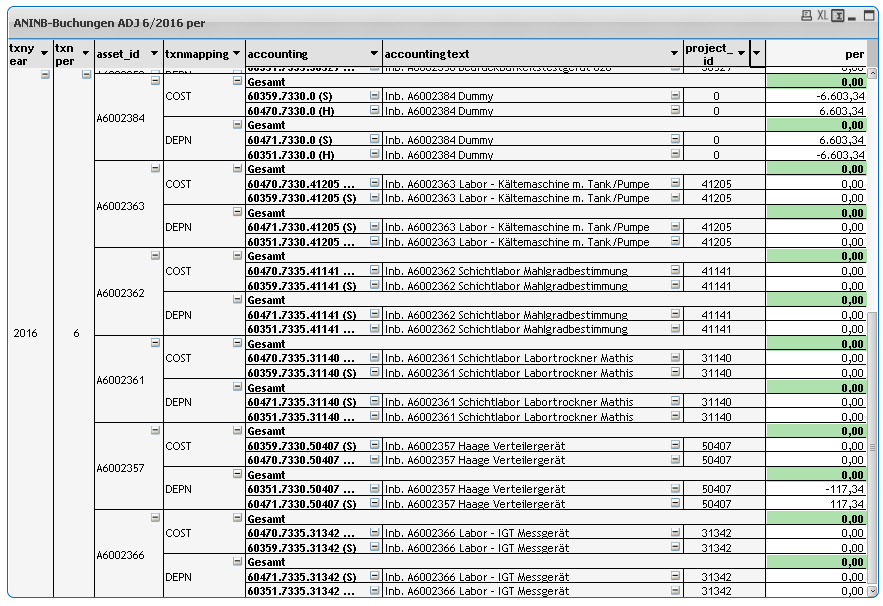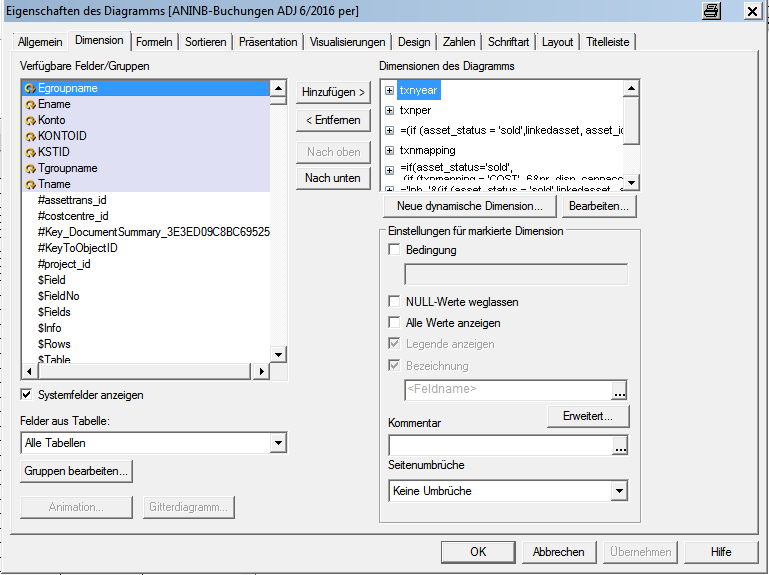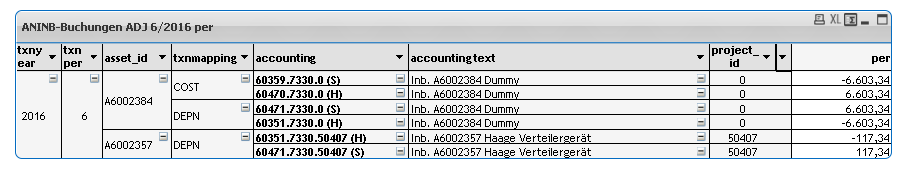# QlikView Creating Analytics

Discussion Board for collaboration related to Creating Analytics for QlikView.

HighlightedNot applicable

## supress lines when calculation is zero (0,00)

Hi,

I have a problem with a Pivot-Table in Qlikview. I have a calculation (please see below), that calculated correct.

My issue is, that I only want to display lines in the Report where the result of the calculation (White lines) <> 0,00.

(green lines are the total) How can I realise this?

This is the calculation of the Field "per"

sum( {\$<book_id={'HGB'},eventmapping={'TRA'}, txnyear={"\$(=\$(Jahr))"}, txnper = {"\$(=\$(Periode))"}>} value*eventflag)
-
sum( {\$<book_id={'IFRS'},eventmapping={'TRA'}, txnyear={"\$(=\$(Jahr))"}, txnper = {"\$(=\$(Periode))"}>} value*eventflag)I tried several things, but nothing works.

It seems to be complicated to supress lines depending on the Expression.

I hope someone have a good idea

Chris

6 Replies
HighlightedMVP

## Re: supress lines when calculation is zero (0,00)

If(Round(

sum( {\$<book_id={'HGB'},eventmapping={'TRA'}, txnyear={"\$(=\$(Jahr))"}, txnper = {"\$(=\$(Periode))"}>} value*eventflag)
-
sum( {\$<book_id={'IFRS'},eventmapping={'TRA'}, txnyear={"\$(=\$(Jahr))"}, txnper = {"\$(=\$(Periode))"}>} value*eventflag)

, 0.01) <> 0,

sum( {\$<book_id={'HGB'},eventmapping={'TRA'}, txnyear={"\$(=\$(Jahr))"}, txnper = {"\$(=\$(Periode))"}>} value*eventflag)
-
sum( {\$<book_id={'IFRS'},eventmapping={'TRA'}, txnyear={"\$(=\$(Jahr))"}, txnper = {"\$(=\$(Periode))"}>} value*eventflag))

HighlightedNot applicable

## Re: supress lines when calculation is zero (0,00)

Hi Sunny,

thanks for you Suggestion. It unfortunately does not work.

the value is not shown anymore but the line is still there.

Chris

HighlightedMVP

## Re: supress lines when calculation is zero (0,00)

Would you be able to share screenshots of dimension tab and presentation tab of your document properties?

HighlightedNot applicable

## Re: supress lines when calculation is zero (0,00)

Hi Sunny,

please find attached the tab-Information. I also included the qwv-file with local month data.

Trigger on opening Shows you directly the Pivot to my questionDIMENSION:

asset_id:  =(if (asset_status = 'sold',linkedasset, asset_id ))

accounting: =if(asset_status='sold',
(
if (txnmapping = 'COST', 6&pr_disp_capnaccount&'.'&kst_id&'.'&project_id&' (S) ', 6&pr_disp_cumdepnaccount&'.'&kst_id&'.'&project_id&' (H) ')),
(
if (txnmapping = 'COST', 6&pr_disp_capnaccount&'.'&kst_id&'.'&project_id&' (H) ', 6&pr_disp_cumdepnaccount&'.'&kst_id&'.'&project_id&' (S) ')))

accounting text: ='Inb. '&(if (asset_status = 'sold',linkedasset , asset_id ))&' '&project_name

project_id: =If( Aggr( Count( Distinct  postrule_id)>1 , txnyear, txnper, project_id), project_id)PRESENTATION:Thanks for you help.

Chris

HighlightedMVP

## Re: supress lines when calculation is zero (0,00)

Was not sure if sharing a sample is a possibility or not. Anyways, is this what you wanted?I just checked 'Suppress Zero-Values' (or Nullwete weglassen in your version) on the presentation tabHighlightedNot applicable

## Re: supress lines when calculation is zero (0,00)

Hi Sunny,

sorry to get back to you so late. Thanks for your answer.

I have only one Problem with "supress Zero values".

The total lines (green ones) are hidden too.

How can I just supress the rows but not the totals?

Chris